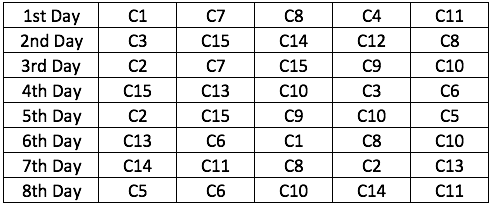Select Page

We will be solving interesting sets as a part of this series LRDI of the Day. I will be posting a video solution by the end of the day. You can keep a track of all the sets by clicking here: LRDI of the Day

#LRDIoftheDay #45

Felonius Gru has 15 different Rubik’s cubes C1 through C15. The cubes are classified into three categories based upon their number of layers. C1, C15, C13 and C4 are of 8 layers each. C6, C2, C7, C9 and C14 are of 6 layers each and the remaining cubes are of 10 layers each. He selects five out of these fifteen cubes on eight consecutive days. On each day he tries to solve the selected cubes but is successful in solving only one of the selected cubes. If he solves a cube on a particular day, that cube is not selected by him on the subsequent days. It is also known that out of the cubes solved by him, the number of cubes of 6 layers is less than the number of cubes of 8 layers.

The following table gives details about the cubes selected by him on each of the given eight days.1. For how many days is it possible to uniquely determine which cube is solved by Gru?

2. If Gru wants the aggregate number of layers of all the cubes that he solves in these 8 days to be the minimum then the number of layers of the cube solved on day 7 is

a. 6

b. 8

c. 10

d. Either 6 or 10

3. After Day 8 Gru finds that the aggregate number of layers of all the cubes that he has solved is more than 64 but not more than 68. Which of the following cubes is definitely solved by Gru?

a. C15

b. C13

c. C10

d. Cannot be determined

4. After Day 8 Gru finds that the aggregate number of layers of all the cubes that he has solved is more than 64 but not more than 68. If C9 is not solved and C8 is solved by Gru, then which of the following can be a cube that is solved by him?

a. C14

b. C5

c. C11

d. C2

The solution

You may go through our popular courses here (at discounted prices): Full LRDI course for CAT 2017 (Rs. 1750 only) | Full VARC course for CAT 2017 (Rs. 1750 only) | Full Quant course for CAT 2017 (Rs. 3500 only) | CAT 2017 full course (Rs. 6000 only) | Current affairs and GK (Rs. 299 only)

error: Content is protected !!